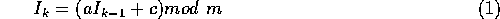# Linear Congruential Generators

One of the most popular methods for generating random numbers is the linear congruential generator. These generators use a method similar to the folding schemes in chaotic maps. The general formula is,The values a, c and m are pre-selected constants. a is known as the multiplier, c is the increment, and m is the modulus.

The quality of the generator is strongly dependent upon the choice of these constants (a significant part of Knuth's chapter on random numbers is dedicated to this topic). The method is appealing however, because once a good set of the parameters is found, it is very easy to program. One fairly obvious goal is to make the period (the time before the generator repeats the sequence) long, this implies that m should be as large as possible. This means that 16 bit random numbers generated by this method have at most a period of 65,536, which is not nearly long enough for serious applications.

The choice of a = 1277, c = 0, m = 131072 looks okay for a while but eventually has problems. A scatter plot for this for 2000 pairs from this generator reveals linear bands emerging from the plot. A random walk plot shows that after a while the slope changes (it should be a constant slope).

The choice of a = 16807, c = 0, m = 2147483647 is a very good set of parameters for this generator. These parameters were published by Park & Miller (1988). This generator often known as the minimal standard random number generator, it is often (but not always) the generator that used for the built in random number function in compilers and other software packages.Next: R250 Up: Random WalksMarkov Chains Previous: Random WalksMarkov Chains

Skip Carter
Tue Jan 9 12:38:54 PST 1996